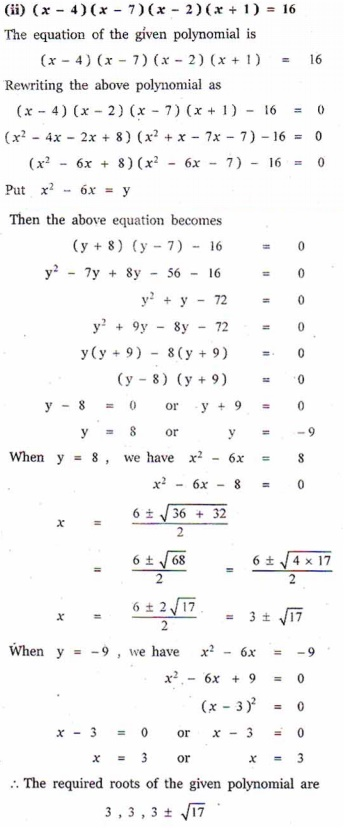Home | | Maths 12th Std | Exercise 3.4: Partly Factored Polynomial

# Exercise 3.4: Partly Factored Polynomial

Maths Book back answers and solution for Exercise questions - Mathematics : Theory of Equations: Partly Factored Polynomial: Exercise Questions with Answer, Solution

EXERCISE 3.4

1. Solve : (i) ( - 5)( - 7)( + 6)( + 4) = 504 (ii) (- 4)(- 7)(- 2)(+1) = 162. Solve : (2-1)(+ 3)(- 2)(2+ 3) + 20 = 0